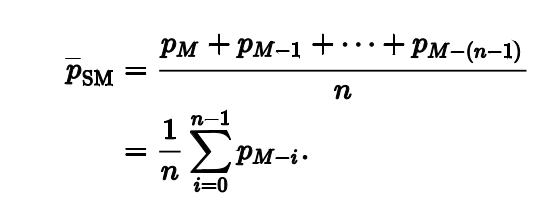• From- To time of the change range
Theme
Bright
Classic black
Pitch black
• Language
• 简体中文
• 한국어
• English
• Tiếng Việt
• 繁体中文
• Español
• Русский язык
• Français
• Deutsch
• Italiano
• Português
• ภาษาไทย
• Indonesia
• Türkçe
• 日本語
Exchange Rate Switch
• CNY
• USD
• KRW
• VND
• EUR
• GBP
• HKD
• JPY
• RUB
• TRY
• INR

### Gate.io Help Center

Customer Support / TicketGate.io > Help Center > Spot & Margin Trade & ETF&CBBC > Quantitative Trading Strategies
##### More article in the group
Dual Moving Average Quantitative Strategy##### Gate.io
Updated at:265 days 11 hours ago
What is the Dual Moving Average?
Moving average is widely used in technical analysis, usually combined with time sequenced data. It is mainly used to smooth out noise of short-term volatility to focus on the long-term trends and cycles. After many years’ development, there are many types of moving averages, the exponential moving average, the weighted moving average and the cumulative moving average. Dual moving averages use the simple moving average (SMA).
Firstly, we calculate the moving average of each trading day based on the close prices of past N days. Then we connect those points to form a line, called N-day moving average. The dual moving average uses two moving average of different terms, 5 day and 60 day, for example.1. About Dual Moving Average Quantitative Strategy
In the dual moving average quantitative strategy, a long term moving average (LTMA) and a short-term moving average (STMA) are used to identify buy and sell opportunities. When the short term moving average breaks above the long term moving average (golden cross), the strategy will go long. When the short-term moving average crosses below the long-term moving average (death crossover), the strategy will go short.

2. Parameter setup
Leverage ratio:
The leverage investor takes. Leverage will be included in calculating the order quantity.
Total Investment:
the total amount of asset an investor put in as margin.
Auto-stop loss ratio:
when the total investment loss reaches the ratio of the total investment, the strategy will close position and exit.
Number of contracts: the number of contracts to be sent to order book under the strategy when a signal is triggered.
Default number of contracts:
here default trading fee is 0.075%
For reverse contract
number of contracts = (margin X last price) / (2* 0.00075 + (1/Leverage) x contract multiplier)
For Direct contracts:
number of contracts = margin/ ((2X0.00075 + (1/leverage)) X Last price X contract multiplier)
The order quantity uses the calculated value or value set by you, whichever is smaller.

Operation example:
After creating an strategy, you can go to My Strategy to view it. When the dual moving average indicator has formed a golden crossover or death crossover, go to Details of the strategy and you can view orders and strategy details.
If you wan to take profit or stop loss, you can click “Close Position” to close an existing position. You can also choose to click “Terminate” to close position and terminate the strategy.

Can't find the answer you want?Submit a Ticket

Popular articles

Articles

Back to top

Gate.io > Help Center > Search result